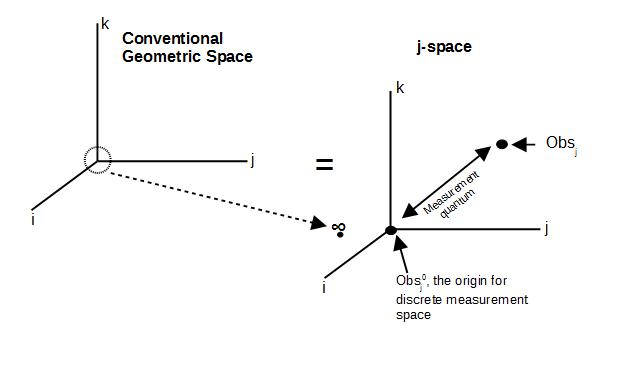# ijspace.org

 The Supersymmetry 18th March 2014              The string theory envisions a super-symmetry which correlates the force and matter in nature. The force is represented by bosons whereas the matter is represented by fermions. Every particle observed in nature should have a super-partner, which LHC is supposed to prove at high energy scales (~TeV). So far we have not succeeded in doing so.     The world we exist in has basic symmetries such as translational symmetry, rotation symmetry, and the symmetry with respect to the inertial frames. If we could add the super-symmetry to these symmetries, we should be able to combine space-time with matter and our description will be complete. However it also means measurements in Planck's domain, which implies the limits of our capabilities. In other words if we can think of the description in terms of discrete measurement space, then the Planck's domain will represent the minimum quantum of discreteness and thus measurements in this region will require maximum capacity of the observer i.e. they will not be easy to perform with our current state of know-how.       Let us step away from the above scenario and try to understand what the concept of symmetry means in terms of the measurements in the discrete measurement space or the j-space. We have earlier discussed the problem of accurate determination of the origin and its implications. The conventional geometric space and its equivalent discrete measurement space is shown below.The observer-defined-origin of the discrete measurement space represents the maximum capacity of the observer Obsj, the observer in j-space. Therefore the earliest symmetry we can introduce,  is the symmetry to accurately determine the origin in terms of the observer capacity. We do this by allowing another observer, Obsj0 which has identical capacity to Obsj and it is used as the reference.     Next we provide the description with respect to the defined-origin which has an implicit symmetry we called Virtual Twin Symmetry or VTS. The quantum nature of the space is quite evident. The description of the nature as observer Obsj perceives it, is not assumed to be absolute but instead limited by the maximum of the observer's (Obsj's), capacity. The true character of Nature remains indeterminate at best for any observer, and hence VTS is a requirement for any description an observer can provide within his or her limited capacity.       It is conceivable therefore that the symmetry leading to the definition of the origin, has to be the symmetry to which other symmetries must converge to. It will lead to chiral characteristics and hence the mass generation. In the following figure Aku and Zörn will agree that the Big-Bang occurred but their description of the Big-Bang and the subsequent chain of events (universe), will differ.Information on www.ijspace.org is licensed under a Creative Commons Attribution 4.0 International License.